# Variable g Pendulum

Mã số: P041213

Nhà sản xuất: AELAB

#### Mục đích:

The period of oscillation of a pendulum is determined mathematically by the length of the pendulum L and the acceleration due to gravity g. The effect of the gravitational acceleration can be demonstrated by tilting the axis of the pendulum so that it is no longer horizontal.

#### 4. On the moon’s surface the “lunar acceleration of gravity” gm is only 16.6% of the earth’s acceleration of gravity g. Calculate the angle α and set it on the device such that the pendulum in the laboratory oscillates with the same oscillation period with which it would oscillate on the moon in a perpendicular position. Compare the measured oscillation period with the calculated one.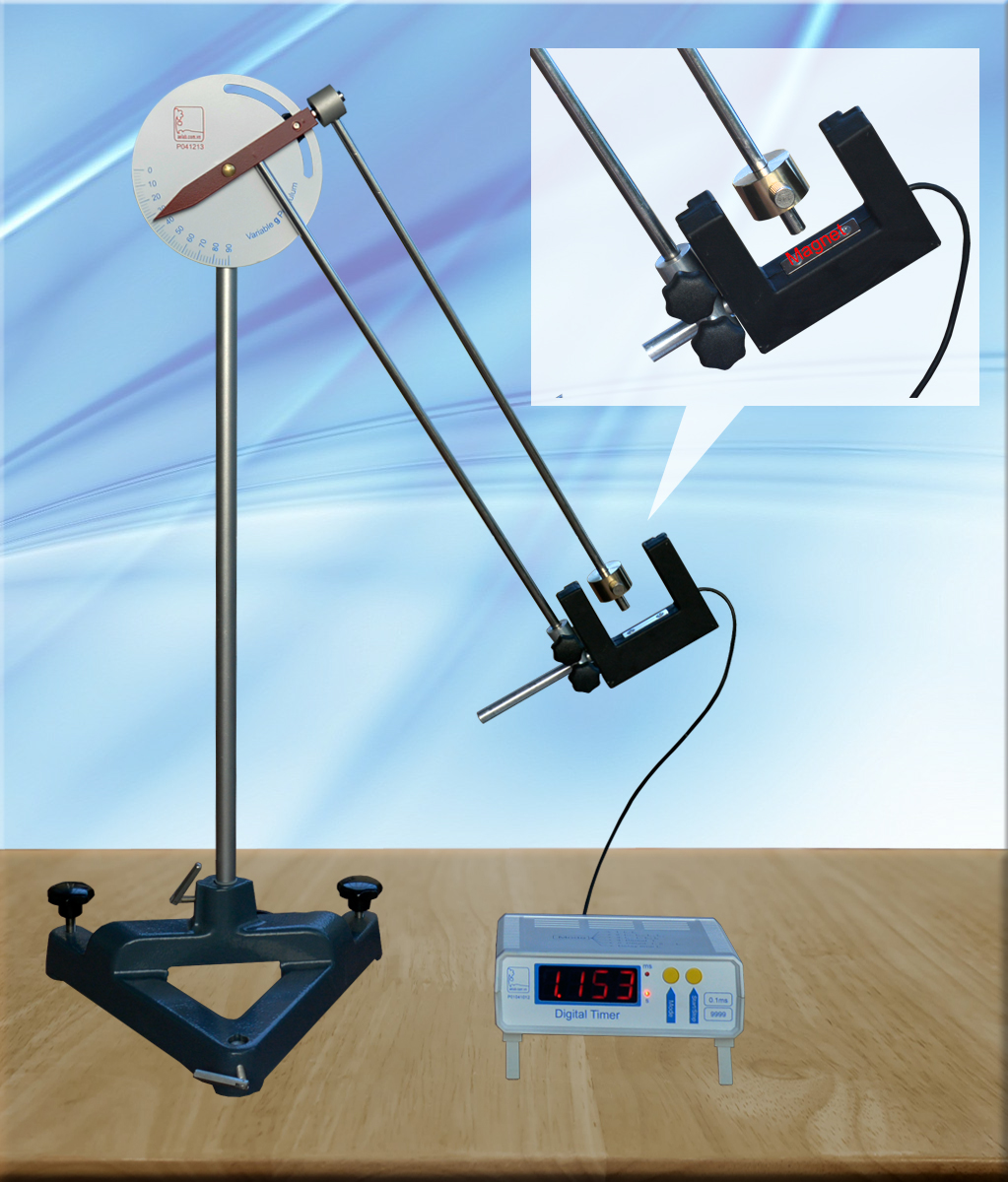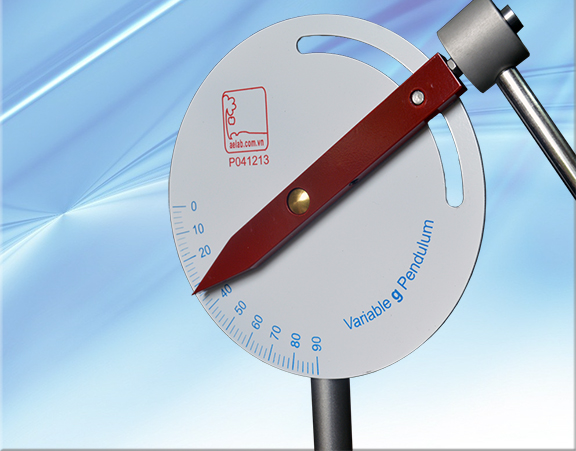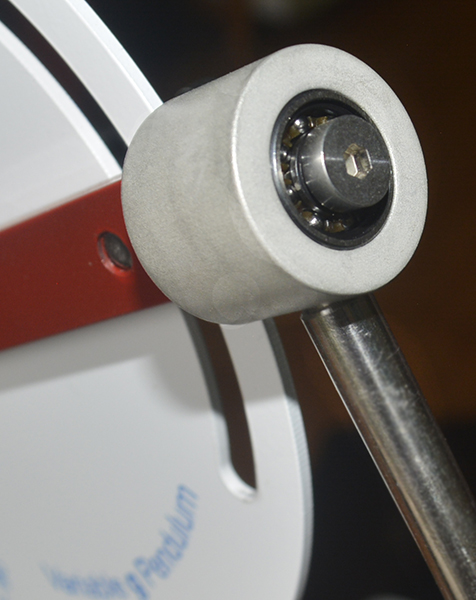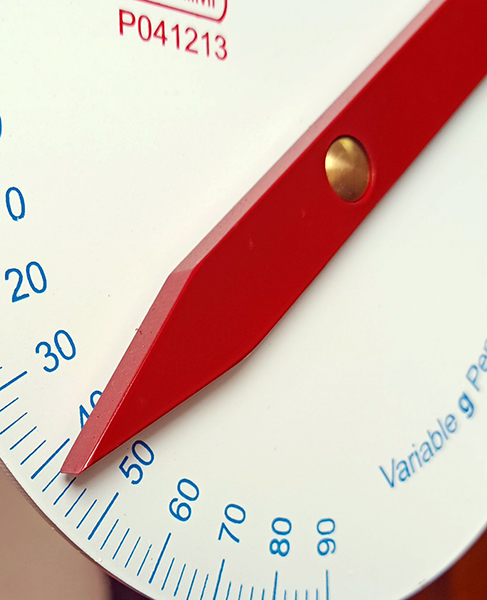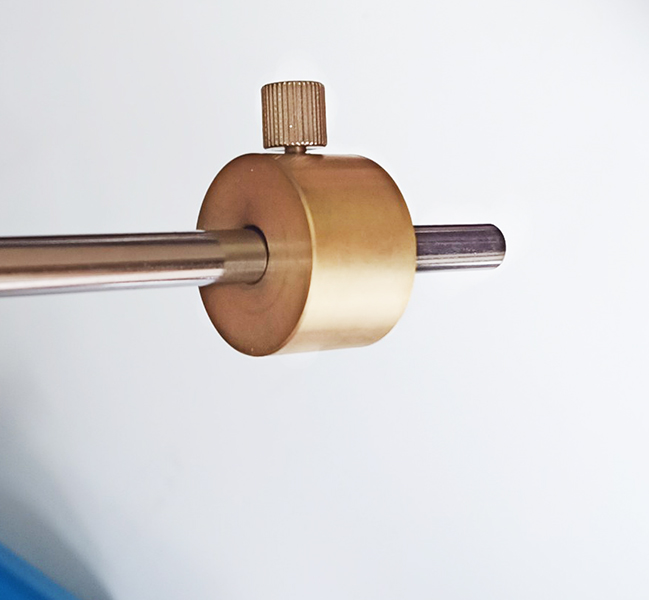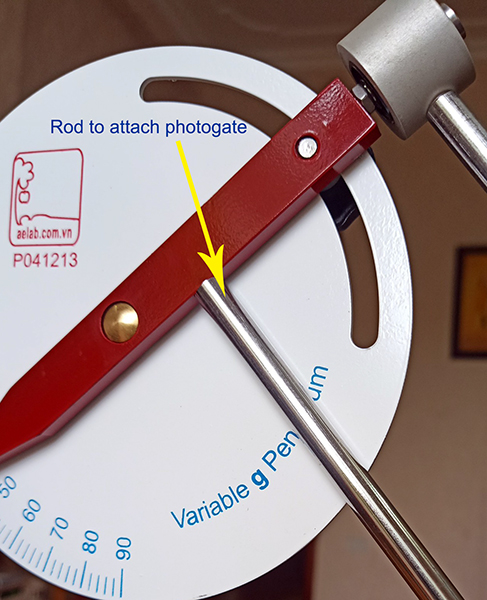When the axis is tilted, the component of the gravitational acceleration g that is parallel to the axis gpar is rendered ineffective by the fact that the axis is fixed (see fig. below). The
remaining component that is effective geff is given by the following equation: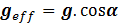(1)

The period of oscillation of the pendulum: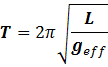(2)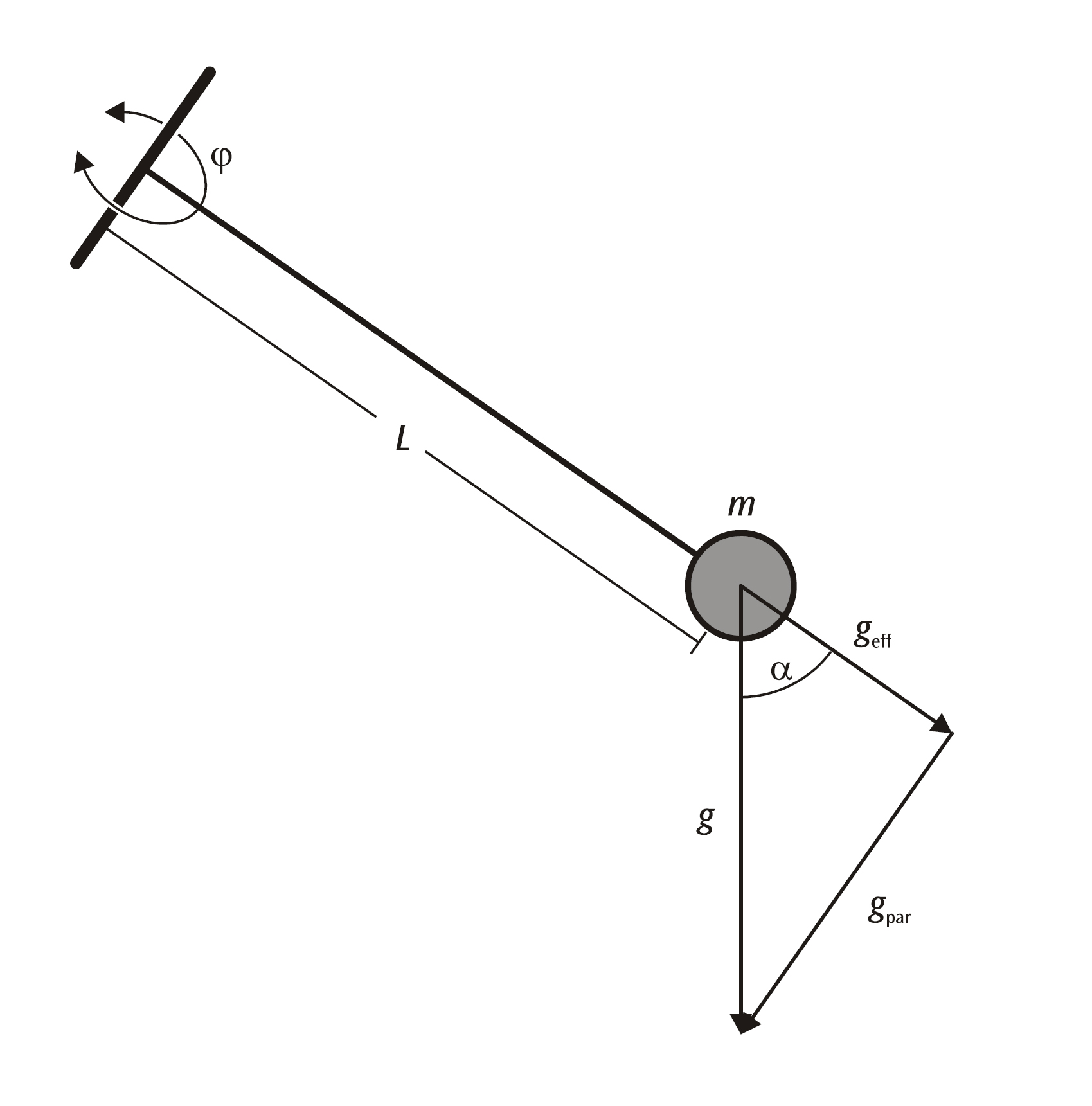Assumption in Hanoi g = 9.81.

With α = 0. Calculation of the effective pendulum length Leff for L = 300mm and L = 445mm.

geff  = g = 9.81

Use formula (2):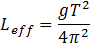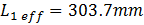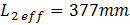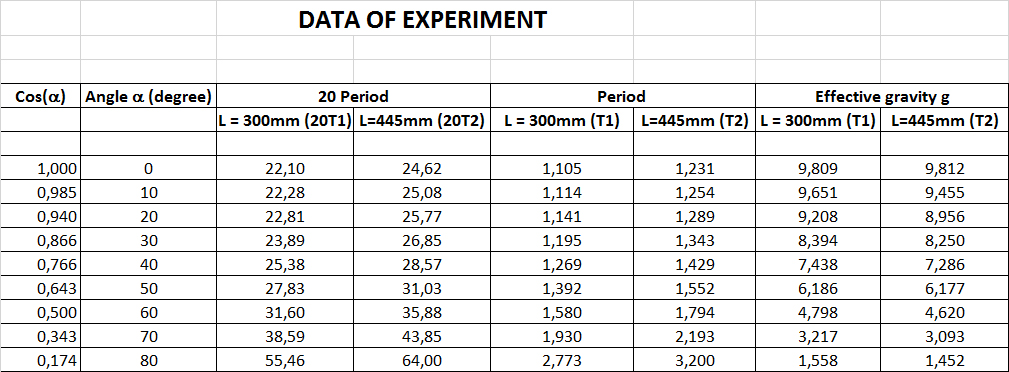Plots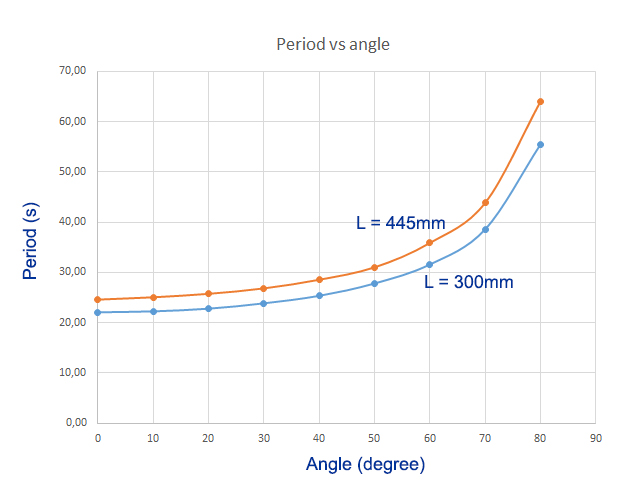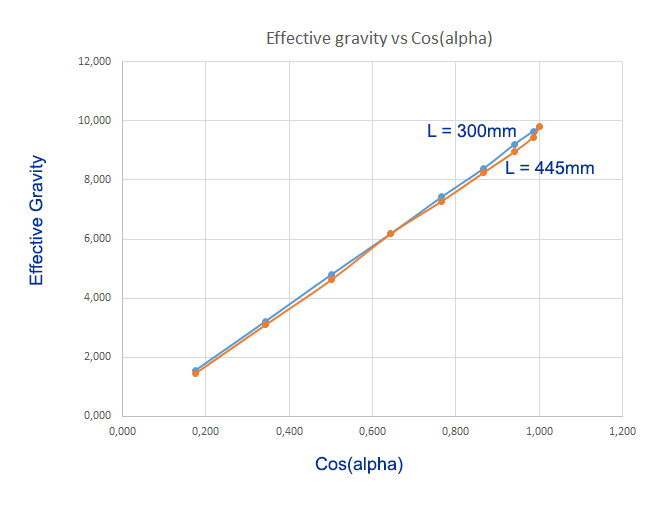gmoon = 16.6%g = 1.625

Based on tables and graphs
We calculate it with a rotation angle of 79 degrees
The pendulum will swing like it is on the moon

Oscillation on Earth and Moon